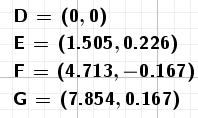# How to find the intersection points between two graphical solutions of the ODESolve command?

jospercomp shared this question 2 years ago

How to find the intersection points between two graphical solutions of the ODESolve command and output are two Locus?{Intersect(f, g)}11

numericalIntegral2=SolveODE(3,4,cos(x),0,0,1,10,0.01)

numericalIntegral2=SolveODE(3,4,sin(x),0,0,1,10,0.01)1

You can find the intersections points these are :1

How converter this command to a Point List? Intersect(Polyline(l1), Polyline(l2))2

{Intersect(f, g)}1

SolveODE(3,4,cos(x),0,0,1,10,0.01)

SolveODE(3,4,sin(x),0,0,1,10,0.01)

l1=First(Element({numericalIntegral1},1),Length(Element({numericalIntegral1},1)))

l2=First(Element({numericalIntegral2},1),Length(Element({numericalIntegral2},1)))

{Intersect(Polyline(l1), Polyline(l2))}